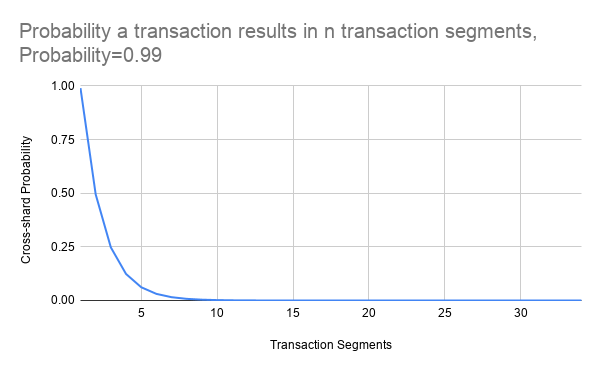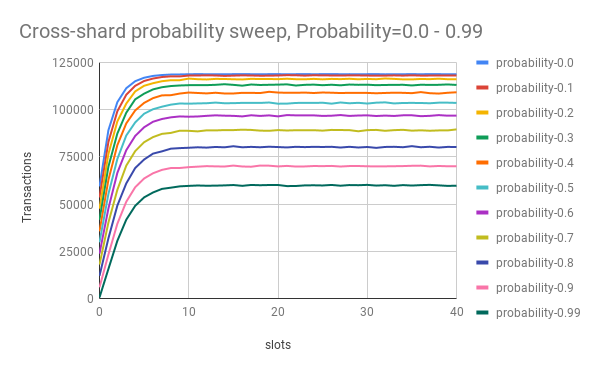# Cross-shard Transaction Probabilistic Simulation

As part of researching cross-shard transactions the TXRX research team has built a cross-shard transaction simulator named Vorpal.

All of the probabilistically generated data can be found here: https://drive.google.com/drive/folders/1sloCwAnJ2Ok2zkuwjtBaFbyZdag-z4Dy?usp=sharing

Throughput is tracked using two metrics, `transactions` and `transaction segments` detailed in this previous research post. Transaction segments are portions of a transaction that result from a cross-shard call, where the transaction is the encapsulation of all the transaction segments.

How cross-shard transaction probabilities are calculated. After each `transaction segment` the cross-shard probability is recomputed resulting in a decaying probability for the encapsulating transaction.

Below is an example of a cross-shard probability calculation at a `probability = 0.99`, and the x axis is the resulting `transaction segments`## Test: Probabilistic cross-shard sweep

This test is a sweep of the `--crossshard` value from `0.0 - 0.99` over multiple simulations. `--crossshard` is the probability a cross-shard call will occur within a transaction.

### Results### Configuration

``````collision_rate	0.0113712

shards	64
slot	12
blocksize	512
witnesssize	256
transactionsize	1
tps	10000
duration	500
probability 0.0 - 0.99
collision	0.01
sweep	FALSE
generate	FALSE
output	None
outputtransactions	None
input	None
``````

### Conclusion

As the probability of a cross-shard transaction increases linearly there is an exponential decrease in transactional throughput. At the maximum value of a cross-shard `probability = 0.99` represents a `~0.503` proportional decrease in transaction throughput.

An average Eth1 transaction contains `~1.33` cross-contract call per transaction.

Assuming shards will have a uniform distribution of contracts the probability of a cross contract call resulting in a cross-shard transaction is `63/64 = ~0.984375` which is very close to the right hand side of this exponential slope. Resulting in `~1.315` cross-shard calls per transaction without any contract modifications or load balancing.

### Recommendations

As a recommendation contract yanking should be implemented as part of the protocol to allow shard balancing.

Cross-shard calls should be economically priced to incentivize the utilization of contract yanking.

### Next Steps

As part of this research the next steps will be to run Eth1 transactions into the simulator to capture non-probabilistic scenarios. Additionally, contract yanking will be tested to detect if there is a improvement in transactional throughput. Investigate in-protocol control loop based contract yanking.

6 Likes

I couldn’t interpret this graph. Maybe I’m missing something. @Joseph Could you please give more details?

1 Like

`probability` is a the probability that an encapsulating `transaction` will result in a cross-shard call, in this instance `0.99`. The simulator uses that value to generate a random number weighted `0.99` for `true` that the `transaction` will result in cross-shard call. These cross-shard calls are called `transaction segments` in the write up.

If the `transaction` results in a cross-shard call the value `probability` is recomputed `probability = probability/2` and applied again to the `transaction`. That gives us the decaying slope of `probability` for a single `transaction`.

Does that help?

Thanks for the details!

An important assumption here is that the probability of x-shard calls is halved in every successive transaction segments, which is a specific usage pattern. An interesting future analysis would be comparing the results for different usage patterns, which are parameterized here by the “probability of the a x-shard call in the next transaction segment” variable. Some probability distributions of interest:

• Uniform
• Exponentially decreasing (already modeled by the current simulation)
• Exponentially increasing: A DoS pattern, where transaction segments are more and more likely to cause a new x-shard call (until the transaction runs out of gas, which can be modeled as a hard limit on # of segments in a transaction)
• Normal(-like) distribution: This would make transactions aim for a average # of segments, after which the probability for new x-shard calls decreases

I am currently analyzing those to create a better simulation, however it would not effect the throughput results in a meaningful way. The current best research can be seen below:

There are actually two probabilities at play in a cross-shard call. The first is the probability that a transaction will result in a cross-contract call where `from != to && from != EOA && to != EOA`. That give the probability that a cross-contract call occurs in a transaction. This data is currently obtainable from Eth1.

The cross-contract call is then computed against a probability of the contract sharing the same shard in a uniform case is which is `63/64 = false`. I am currently enhancing my simulator to account for different non-uniform distributions as you suggested. I am planning a third update on this topic following further analysis.

1 Like